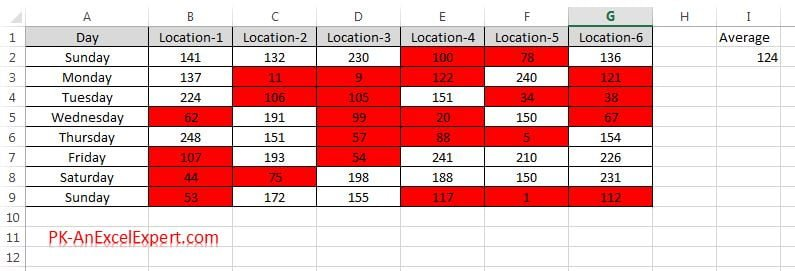# Chapter-9: Format only values that are above or below average

Format only values that are above or below average” rule is used to highlight the excel cells which have the value above/below the average of the conditional formatting range.

#### To apply “Format only values that are above or below average” rule below are the steps

• Select the range on which you want to apply the conditional formatting
• As in below image select “B2:G9”
• Go to Home tab>>Conditional Formatting>>New Rules
• New formatting rule window will be opened.
• Select “Format only values that are above or below average
• Select the above or below (here we are highlighting the cells which have value above the average)
• click on Format button.
• Format Cells window will be opened.
• Give the formatting which you want to apply on the excel cells
• Click on OK button to apply this conditional formatting.
• Data set with this conditional formatting will look like below image.Data Sets after applying the conditional formatting of Above Average

### Highlight the cells which are below average

• Select below in the drop down.
• Give the format whatever you want to show.
• Click on OK button to apply this conditional formatting.
• Data set with this conditional formatting will look like below image.Data Sets after applying the conditional formatting of Below Average

### You can apply this rule the cells which are from average

• above
• below
• equal or above
• equal or below
• 1 std dev above
• 1 std dev below
• 2 std dev above
• 2 std dev below
• 3 std dev above
• 3 std dev below